# 10 multiple choice and 5 short answer calculus questions

1.

Use your graphing calculator to evaluate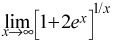. (5 points)

 0 e π 1

2.

Describe the discontinuity for the function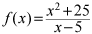. (5 points)

 There is a hole at x = -25. There is a removable discontinuity at x = 5. There is a vertical asymptote at x = 5. There is no discontinuity at x = 5.

3.

Find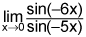. (5 points)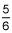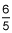Does not exist 0

4.

Evaluate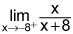. (5 points)

 ∞ -∞ 0 -8

5.

Evaluate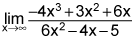. (5 points)

 -2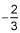0 -∞

6.

Which of the following is the graph of which function has y = -3 as an asymptote? (5 points)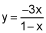y = 3ln(x + 1)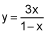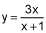7.

If is continuous at x = -4, find f(-4). (5 points)

 4 -4 8 -8

8.

Where is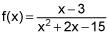discontinuous? (5 points)

 f(x) is continuous everywhere x = 3 and x = -5 x = -5 x = 3

9.

If f(x) is discontinuous, determine the reason.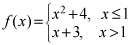(5 points)

 f(x) is continuous for all real numbers The limit as x approaches 1 does not exist f(1) does not equal the limit as x approaches 1 f(1) is not defined

10.

If f(x) is a continuous function defined for all real numbers, f(-10) = -2, f(-8) = 5, and f(x) = 0 for one and only one value of x, then which of the following could be that x value? (5 points)

 -7 -9 0 2

1.

Use the graph below to list the x value(s) where the limits as x approaches from the left and right of those integer values(s) are not equal.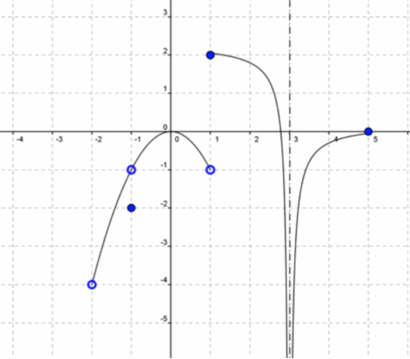(10 points)

2.

Find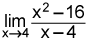. You must show your work or explain your work in words. (10 points)

3.

Find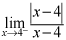. You must show your work or explain your work in words. (10 points)

4.

A ball’s position, in meters, as it travels every second is represented by the position function s(t) = 4.9t2 + 350.

What is the velocity of the ball after 2 seconds?